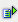The function proper (string) converts the first character of a word to upper case. The start of a word is after each non-alphabetical character.

All other characters are set to lower case.

Syntax: =proper(string)Example Cell A1 = AbCdEf =lower(A1) is abcdef Cell A1 = AbCdEf =lower(A1) is abcdef Cell A1 = AbCdEf =lower(A1) is abcdef